Published: 15 November 2015

# Investigation on dynamics of a three-directional coupled vehicle-road system

Shaohua Li1
Shaopu Yang2
1, 2Shijiazhuang Tiedao University, Shijiazhuang, China
Corresponding Author:
Shaohua Li
Views 75

## 1. Introduction

However, vehicle dynamics and road dynamics are two separate subjects at present. In these two subjects, the vehicle-road interaction is investigated using different models and methods, and a lot of evaluation index of road damage and design rules of vehicle and road parameters have been proposed and concluded.

This work tries to connect the vehicle and road by vertical, lateral and longitudinal tire forces, and put forward the methodology of three-directional (3D) coupled vehicle-road system modeling and simulating. Based on a 3D vehicle-road coupled model, the differences of 3D coupled, vertical coupled and uncoupled model are compared for pavement displacements and vehicle responses. The effects of vehicle maneuver parameters and road surface properties on 3D vehicle-road interaction are also analyzed. The proposed 3D-coupled vehicle-road model and the simulation method are able to research the vehicle-road interaction and will be beneficial to vehicle and road dynamics study.

## 2. Modeling the three-directional coupled vehicle-road system

A three-directional (3D) coupled vehicle-road system is proposed in this work as shown in Fig. 1. A 23-DOF vehicle and a double-layer rectangular thin plate on viscoelastic foundation with four simply supported boundaries are employed to model vehicle and pavement. The upper layer of the plate models asphalt topping, the lower layer models base course, and the viscoelastic foundation stands for subgrade of the road. The material of topping and base course is assumed to be isotropy and elastic. The vehicle is able to moving on the road in different maneuver conditions, such as straight-line running at a constant speed, braking and turning. The origin of vehicle coordinate system ($x$, $y$, $z$) is located in the intersection between the roll axle and the vertical line passing vehicle center of gravity. $\psi$ is the vehicle’s yaw angle which describe the orientation of the vehicle. The static ground coordinate system ($X$, $Y$, $Z$) is also shown in Fig. 1, taking the stress neutral layer of pavement as coordinate axial $X$, the lateral direction as coordinate axial $Y$ and the vertical direction as coordinate axial $Z$.

Fig. 1The 3D coupled vehicle-road model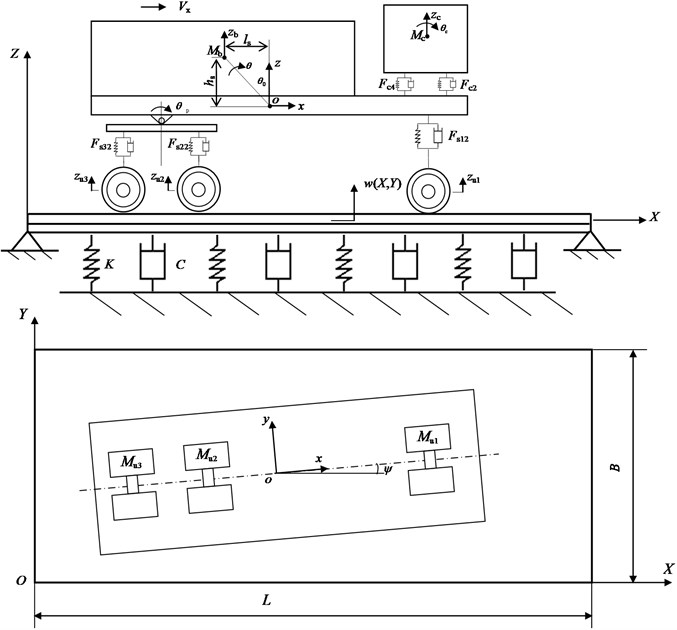### 2.1. Equations of motion for the vehicle

A nonlinear full-body model with 23-DOF for a three-axial heavy-duty truck is presented, as shown in Fig. 2. The vehicle is front wheel steered and rear wheel driven. $x$, $y$, $\psi$ represent the longitudinal and lateral displacements and the yaw angle of the full vehicle respectively. ${z}_{c}$, ${\theta }_{c}$, ${\varphi }_{c}$, ${z}_{b}$, $\theta$, $\varphi$ stand for the vertical, pitch and roll displacements of driver cab and vehicle body. ${z}_{ui}$, ${\varphi }_{ui}$ ($i=$1,…, 3) denote the vertical and roll displacements of three wheel axles. ${\theta }_{p1}$, ${\theta }_{p2}$ are the pitch angles of the left and right balancing pole on rear suspension. $M$, ${M}_{b}$, ${M}_{c}$ denote the masses of full vehicle, vehicle body and driver cab respectively. ${h}_{s}$, ${l}_{s}$ are the vertical and longitudinal distance from the sprung mass center of gravity to the coordinate origin. ${d}_{t1}$, ${d}_{t2}$, ${d}_{t3}$ are the front and rear wheel track width. ${d}_{s1}$, ${d}_{s2}$, ${d}_{s3}$ are the lateral distance between left and right spring on front or rear suspension.

The movements of the heavy-duty vehicle are coupled with each other greatly. Eqs. (1)-(3) give the longitudinal, lateral and yaw dynamics of the full vehicle. Eqs. (4)-(6) give the vertical, roll and pitch dynamics of the sprung mass:

1
$M\left(\stackrel{¨}{x}-\stackrel{˙}{y}\stackrel{˙}{\psi }\right)+{M}_{b}{\stackrel{˙}{z}}_{b}\stackrel{˙}{\theta }+{M}_{b}{h}_{s}\stackrel{¨}{\theta }-{M}_{b}{l}_{s}\left({\stackrel{˙}{\psi }}^{2}+{\stackrel{˙}{\theta }}^{2}\right)=\left({F}_{tx11}+{F}_{tx12}\right)\mathrm{c}\mathrm{o}\mathrm{s}\delta$
$\mathrm{}\mathrm{}\mathrm{}\mathrm{}\mathrm{}\mathrm{}-\left({F}_{ty11}+{F}_{ty12}\right)\mathrm{s}\mathrm{i}\mathrm{n}\delta +\sum _{i=2}^{3}\sum _{j=1}^{2}{F}_{txij},$
2
$M\left(\stackrel{¨}{y}+\stackrel{˙}{x}\stackrel{˙}{\psi }\right)-{M}_{b}{\stackrel{˙}{z}}_{b}\stackrel{˙}{\phi }-{M}_{b}{h}_{s}\stackrel{¨}{\phi }=\left({F}_{tx11}+{F}_{tx12}\right)\mathrm{s}\mathrm{i}\mathrm{n}\delta$
$\mathrm{}\mathrm{}\mathrm{}\mathrm{}\mathrm{}\mathrm{}\mathrm{}+\left({F}_{ty11}+{F}_{ty12}\right)\mathrm{c}\mathrm{o}\mathrm{s}\delta +\sum _{i=2}^{3}\sum _{j=1}^{2}{F}_{tyij},$
3
${I}_{z}\stackrel{¨}{\psi }+2{I}_{zb}\stackrel{˙}{\phi }\stackrel{˙}{\theta }-\left({I}_{bxz}+{M}_{b}{l}_{s}{h}_{s}\right)\stackrel{¨}{\phi }-{M}_{b}{l}_{s}\left(\stackrel{¨}{y}+\stackrel{˙}{x}\stackrel{˙}{\psi }-{\stackrel{˙}{z}}_{b}\stackrel{˙}{\phi }\right)={l}_{1}\left[\left({F}_{tx11}+{F}_{tx12}\right)\mathrm{s}\mathrm{i}\mathrm{n}\delta$
$\mathrm{}\mathrm{}\mathrm{}\mathrm{}\mathrm{}\mathrm{}+\left({F}_{ty11}+{F}_{ty12}\right)\mathrm{c}\mathrm{o}\mathrm{s}\delta \right]-\left({l}_{2}-\frac{{l}_{3}}{2}\right)\mathrm{}\sum _{j=1}^{2}{F}_{ty2j}-\left({l}_{2}+\frac{{l}_{3}}{2}\right)\mathrm{}\sum _{j=1}^{2}{F}_{ty3j}$
$\mathrm{}\mathrm{}\mathrm{}\mathrm{}\mathrm{}\mathrm{}+\frac{{d}_{t1}}{2}\left[\left(-{F}_{tx11}+{F}_{tx12}\right)\mathrm{c}\mathrm{o}\mathrm{s}\delta +\left({F}_{ty11}-{F}_{ty12}\right)\mathrm{s}\mathrm{i}\mathrm{n}\delta \right]$
$\mathrm{}\mathrm{}\mathrm{}\mathrm{}\mathrm{}\mathrm{}+\frac{{d}_{t2}}{2}\left(-{F}_{ty21}+{F}_{ty22}-{F}_{ty21}+{F}_{ty22}\right)+\sum _{i=1}^{3}\sum _{j=1}^{2}{T}_{zij},$
4
${M}_{b}\left({\stackrel{¨}{z}}_{b}-\stackrel{˙}{x}\stackrel{˙}{\theta }+\stackrel{˙}{y}\stackrel{˙}{\phi }\right)-{M}_{b}{h}_{s}\left({\stackrel{˙}{\theta }}^{2}+{\stackrel{˙}{\phi }}^{2}\right)-{M}_{b}{l}_{s}\stackrel{¨}{\theta }-\left({F}_{c1}+{F}_{c2}+{F}_{c3}+{F}_{c4}\right)$
$\mathrm{}\mathrm{}\mathrm{}\mathrm{}\mathrm{}\mathrm{}+\left({F}_{s11}+{F}_{s12}+{F}_{s21}+{F}_{s22}+{F}_{s31}+{F}_{s32}\right)=-{M}_{b}g,$
5
$\left({M}_{b}{{h}_{s}}^{2}+{I}_{bx}\right)\stackrel{¨}{\phi }-\left({I}_{bxz}+{M}_{b}{l}_{s}{h}_{s}\right)\left(\stackrel{¨}{\psi }+2\stackrel{˙}{\phi }\stackrel{˙}{\theta }\right)-{M}_{b}{h}_{s}\left(\stackrel{¨}{y}+\stackrel{˙}{x}\stackrel{˙}{\psi }-{\stackrel{˙}{z}}_{b}\stackrel{˙}{\phi }\right)$
$\mathrm{}\mathrm{}\mathrm{}\mathrm{}\mathrm{}\mathrm{}+\left({F}_{c2}+{F}_{c4}-{F}_{c1}-{F}_{c3}\right)\frac{{d}_{c}}{2}+\left({F}_{s11}-{F}_{s12}\right)\frac{{d}_{s1}}{2}+\left({F}_{s21}-{F}_{s22}\right)\frac{{d}_{s2}}{2}$
$\mathrm{}\mathrm{}\mathrm{}\mathrm{}\mathrm{}\mathrm{}+\left({F}_{s31}-{F}_{s32}\right)\frac{{d}_{s3}}{2}=\left({z}_{b}-{z}_{t11}+{R}_{11}\right)\left({F}_{tx11}\mathrm{s}\mathrm{i}\mathrm{n}\delta +{F}_{ty11}\mathrm{c}\mathrm{o}\mathrm{s}\delta \right)$
$\mathrm{}\mathrm{}\mathrm{}\mathrm{}\mathrm{}\mathrm{}+\left({z}_{b}-{z}_{t12}+{R}_{12}\right)\left({F}_{tx12}\mathrm{s}\mathrm{i}\mathrm{n}\delta +{F}_{ty12}\mathrm{c}\mathrm{o}\mathrm{s}\delta \right)+\sum _{i=2}^{3}\sum _{j=1}^{2}{F}_{tyij}\left({z}_{b}-{z}_{tij}+{R}_{ij}\right),$
6
$\left({M}_{b}{h}_{s}^{2}+{I}_{by}\right)\stackrel{¨}{\theta }-{M}_{b}{h}_{s}^{}{l}_{s}\left({\stackrel{˙}{\psi }}^{2}+{\stackrel{˙}{\theta }}^{2}\right)+{M}_{b}{h}_{s}\left(\stackrel{¨}{x}-\stackrel{˙}{y}\stackrel{˙}{\psi }+{\stackrel{˙}{z}}_{b}\stackrel{˙}{\theta }\right)-{M}_{b}{l}_{s}\left({\stackrel{¨}{z}}_{b}+g-\stackrel{˙}{x}\stackrel{˙}{\theta }+\stackrel{˙}{y}\stackrel{˙}{\phi }\right)$
$\mathrm{}\mathrm{}\mathrm{}\mathrm{}\mathrm{}\mathrm{}+{M}_{b}{l}_{{\mathrm{}}_{s}}^{2}\stackrel{¨}{\theta }+\left({F}_{c1}+{F}_{c2}\right)\left({l}_{4}+{l}_{5}\right)+\left({F}_{c3}+{F}_{c4}\right)\left({l}_{4}-{l}_{6}\right)-\left({F}_{s11}+{F}_{s12}\right){l}_{1}$
$\mathrm{}\mathrm{}\mathrm{}\mathrm{}\mathrm{}\mathrm{}+\left({F}_{s21}+{F}_{s22}+{F}_{s31}+{F}_{s32}\right){l}_{2}=\left({z}_{b}-{z}_{t11}+{R}_{11}\right)\left({F}_{tx11}\mathrm{c}\mathrm{o}\mathrm{s}\delta -{F}_{ty11}\mathrm{s}\mathrm{i}\mathrm{n}\delta \right)$
$\mathrm{}\mathrm{}\mathrm{}\mathrm{}\mathrm{}\mathrm{}+\left({z}_{b}-{z}_{t12}+{R}_{12}\right)\left({F}_{tx12}\mathrm{c}\mathrm{o}\mathrm{s}\delta -{F}_{ty12}\mathrm{s}\mathrm{i}\mathrm{n}\delta \right)+\sum _{i=2}^{3}\sum _{j=1}^{2}{F}_{txij}\left({z}_{b}-{z}_{tij}+{R}_{ij}\right),$

where $\delta$, ${T}_{zij}$ and ${R}_{ij}$ are the steering angle of front wheel, the tire aligning torque, and the tire effective radius respectively. ${F}_{cs}$ ($s=$1,…, 4) denotes the suspension forces between driver cab and vehicle body and can be expressed as:

7
$\left\{\begin{array}{l}{F}_{c1}={K}_{c1}\left({z}_{c}-{\theta }_{c}{l}_{5}-{z}_{b}+\left(\theta -{\theta }_{0}\right)\left({l}_{4}+{l}_{5}\right)-\frac{\left(\phi -{\phi }_{c}\right){d}_{c}}{2}\right)\\ \mathrm{}\mathrm{}\mathrm{}\mathrm{}\mathrm{}\mathrm{}\mathrm{}+{C}_{c1}\left({\stackrel{˙}{z}}_{c}-{\stackrel{˙}{\theta }}_{c}{l}_{5}-{\stackrel{˙}{z}}_{b}+\stackrel{˙}{\theta }\left({l}_{4}+{l}_{5}\right)-\frac{\left(\stackrel{˙}{\phi }-{\stackrel{˙}{\phi }}_{c}\right){d}_{c}}{2}\right),\\ {F}_{c2}={K}_{c2}\left({z}_{c}-{\theta }_{c}{l}_{5}-{z}_{b}+\left(\theta -{\theta }_{0}\right)\left({l}_{4}+{l}_{5}\right)+\frac{\left(\phi -{\phi }_{c}\right){d}_{c}}{2}\right)\\ \mathrm{}\mathrm{}\mathrm{}\mathrm{}\mathrm{}\mathrm{}\mathrm{}+{C}_{c2}\left({\stackrel{˙}{z}}_{c}-{\stackrel{˙}{\theta }}_{c}{l}_{5}-{\stackrel{˙}{z}}_{b}+\stackrel{˙}{\theta }\left({l}_{4}+{l}_{5}\right)+\frac{\left(\stackrel{˙}{\phi }-{\stackrel{˙}{\phi }}_{c}\right){d}_{c}}{2}\right),\\ {F}_{c3}={K}_{c3}\left({z}_{c}+{\theta }_{c}{l}_{6}-{z}_{b}+\left(\theta -{\theta }_{0}\right)\left({l}_{4}-{l}_{6}\right)-\frac{\left(\phi -{\phi }_{c}\right){d}_{c}}{2}\right)\\ \mathrm{}\mathrm{}\mathrm{}\mathrm{}\mathrm{}\mathrm{}\mathrm{}+{C}_{c3}\left({\stackrel{˙}{z}}_{c}+{\stackrel{˙}{\theta }}_{c}{l}_{6}-{\stackrel{˙}{z}}_{b}+\stackrel{˙}{\theta }\left({l}_{4}-{l}_{6}\right)-\frac{\left(\stackrel{˙}{\phi }-{\stackrel{˙}{\phi }}_{c}\right){d}_{c}}{2}\right),\\ {F}_{c4}={K}_{c4}\left({z}_{c}+{\theta }_{c}{l}_{6}-{z}_{b}+\left(\theta -{\theta }_{0}\right)\left({l}_{4}-{l}_{6}\right)+\frac{\left(\phi -{\phi }_{c}\right){d}_{c}}{2}\right)\\ \mathrm{}\mathrm{}\mathrm{}\mathrm{}\mathrm{}\mathrm{}\mathrm{}+{C}_{c4}\left({\stackrel{˙}{z}}_{c}+{\stackrel{˙}{\theta }}_{c}{l}_{6}-{\stackrel{˙}{z}}_{b}+\stackrel{˙}{\theta }\left({l}_{4}-{l}_{6}\right)+\frac{\left(\stackrel{˙}{\phi }-{\stackrel{˙}{\phi }}_{c}\right){d}_{c}}{2}\right).\end{array}\right\$

For this heavy-duty vehicle, two hydraulic dampers are fixed on the left and right front suspension and the tandem balanced suspension doesn’t have any damper. The damper in vehicle suspension is one of the major factors influencing the vehicle handling and ride comfort and shows apparent nonlinearity, asymmetry and hysteresis. Many dynamic models for vehicle suspension damper have been proposed, including the parametric model , the equivalent parametric model [34, 35] and the fitted model [36, 37]. The fitted model is quite suitable to modeling the ascertained damper and the function of restoring force relate to velocity are fitted by the test data. In this work, the fitted model is used. The damping force of the damper on front axle can be described by a nonlinear segmented model:

8
${F}_{d}=\left\{\begin{array}{ll}{F}_{01}\mathrm{s}\mathrm{g}\mathrm{n}\left({v}_{d}\right),& {v}_{d}>{v}_{\mathrm{l}\mathrm{i}\mathrm{m}1},\\ C\left(1+\beta \mathrm{s}\mathrm{g}\mathrm{n}\left({v}_{d}\right)\right){v}_{d}{\left|{v}_{d}\right|}^{n},& {v}_{\mathrm{l}\mathrm{i}\mathrm{m}2}\le {v}_{d}\le {v}_{\mathrm{l}\mathrm{i}\mathrm{m}1},\\ {F}_{02}\mathrm{s}\mathrm{g}\mathrm{n}\left({v}_{d}\right),& {v}_{d}<{v}_{\mathrm{l}\mathrm{i}\mathrm{m}2},\end{array}\right\$

where ${v}_{d}$, $C$, $\beta$ and $n$ are the relative velocity of cylinder and plunger, the damping coefficient, the asymmetry ratio and the exponent respectively. ${F}_{01}$, ${F}_{02}$, ${v}_{\mathrm{l}\mathrm{i}\mathrm{m}1}$ and ${v}_{\mathrm{l}\mathrm{i}\mathrm{m}2}$ are the damping force and relative velocity when the damper reaching saturation in tension or compression process. Eq. (8) is composed of three equations and modeled from the test data . The first and third equations are dry friction damping models, which define the damping forces when the damper plunger arrives at the above or below limit position. The second equation describes the hysteresis viscous damping force when the plunger moves between the two limit positions. Here, $C=$30893, $\beta =\mathrm{}$0.56, $n=$0.16, ${F}_{01}=$4119 N, ${F}_{02}=$726 N, ${v}_{\mathrm{l}\mathrm{i}\mathrm{m}1}=$0.12 m/s, ${v}_{\mathrm{l}\mathrm{i}\mathrm{m}2}=$0.08 m/s. These parameters are obtained by fitting the measured data of the damper’s dynamic test. The test’s description and the related results were given by the author’s another work .

Fig. 2The full-body heavy vehicle model with 23-DOF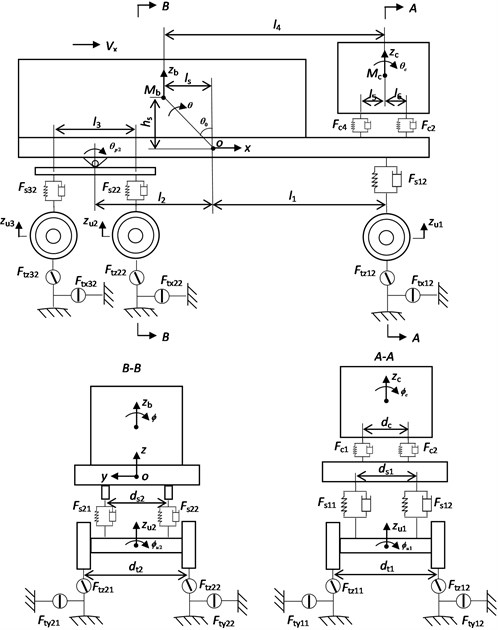Using model Eq. (8) to calculate the damping force, the front suspension forces are expressed by:

9
${F}_{s11}={K}_{s11}\left({z}_{b}-\left(\theta -{\theta }_{0}\right){l}_{1}-{z}_{u1}+\frac{\left(\phi -{\phi }_{u1}\right){d}_{s1}}{2}\right)+{F}_{d11},$
${F}_{s12}={K}_{s12}\left({z}_{b}-\left(\theta -{\theta }_{0}\right){l}_{1}-{z}_{u1}-\frac{\left(\phi -{\phi }_{u1}\right){d}_{s1}}{2}\right)+{F}_{d12},$

where ${F}_{d11}$, ${F}_{d12}$ are the left and right damping force of front suspension. The relative velocities of left and right damper are obtained by ${v}_{d1}=\left({\stackrel{˙}{z}}_{b}-\stackrel{˙}{\theta }{l}_{1}-{\stackrel{˙}{z}}_{u1}+\left(\stackrel{˙}{\phi }-{\stackrel{˙}{\phi }}_{u1}\right){d}_{s1}/2\right)$ and ${v}_{d2}=\left({\stackrel{˙}{z}}_{b}-\stackrel{˙}{\theta }{l}_{1}-{\stackrel{˙}{z}}_{u1}-\left(\stackrel{˙}{\phi }-{\stackrel{˙}{\phi }}_{u1}\right){d}_{s1}/2\right)$.

In order to represent the frictional property of leaf spring, the damping forces of tandem balanced suspension are modeled linearly. The suspension forces between middle or rear axle and vehicle body are expressed by:

10
$\left\{\begin{array}{l}{F}_{s21}={K}_{s21}\left({z}_{b}+\left(\theta -{\theta }_{0}\right){l}_{2}-\frac{{\theta }_{p1}{l}_{3}}{2}-{z}_{u2}+\frac{\left(\phi -{\phi }_{u2}\right){d}_{s2}}{2}\right)\\ \mathrm{}\mathrm{}\mathrm{}\mathrm{}\mathrm{}\mathrm{}\mathrm{}+{C}_{s21}\left({\stackrel{˙}{z}}_{b}+\stackrel{˙}{\theta }{l}_{2}-\frac{{\stackrel{˙}{\theta }}_{p1}{l}_{3}}{2}-{\stackrel{˙}{z}}_{u2}+\frac{\left(\stackrel{˙}{\phi }-{\stackrel{˙}{\phi }}_{u2}\right){d}_{s2}}{2}\right),\\ {F}_{s22}={K}_{s22}\left({z}_{b}+\left(\theta -{\theta }_{0}\right){l}_{2}-\frac{{\theta }_{p2}{l}_{3}}{2}-{z}_{u2}-\frac{\left(\phi -{\phi }_{u2}\right){d}_{s2}}{2}\right)\\ \mathrm{}\mathrm{}\mathrm{}\mathrm{}\mathrm{}\mathrm{}\mathrm{}+{C}_{s22}\left({\stackrel{˙}{z}}_{b}+\stackrel{˙}{\theta }{l}_{2}-\frac{{\stackrel{˙}{\theta }}_{p2}{l}_{3}}{2}-{\stackrel{˙}{z}}_{u2}-\frac{\left(\stackrel{˙}{\phi }-{\stackrel{˙}{\phi }}_{u2}\right){d}_{s2}}{2}\right),\\ {F}_{s31}={K}_{s31}\left({z}_{b}+\left(\theta -{\theta }_{0}\right){l}_{2}+\frac{{\theta }_{p1}{l}_{3}}{2}-{z}_{u3}+\frac{\left(\phi -{\phi }_{u3}\right){d}_{s3}}{2}\right)\\ \mathrm{}\mathrm{}\mathrm{}\mathrm{}\mathrm{}\mathrm{}\mathrm{}+{C}_{s31}\left({\stackrel{˙}{z}}_{b}+\stackrel{˙}{\theta }{l}_{2}+\frac{{\stackrel{˙}{\theta }}_{p1}{l}_{3}}{2}-{\stackrel{˙}{z}}_{u3}+\frac{\left(\stackrel{˙}{\phi }-{\stackrel{˙}{\phi }}_{u3}\right){d}_{s3}}{2}\right),\\ {F}_{s32}={K}_{s32}\left({z}_{b}+\left(\theta -{\theta }_{0}\right){l}_{2}+\frac{{\theta }_{p2}{l}_{3}}{2}-{z}_{u3}-\frac{\left(\phi -{\phi }_{u3}\right){d}_{s3}}{2}\right)\\ \mathrm{}\mathrm{}\mathrm{}\mathrm{}\mathrm{}\mathrm{}\mathrm{}+{C}_{s32}\left({\stackrel{˙}{z}}_{b}+\stackrel{˙}{\theta }{l}_{2}+\frac{{\stackrel{˙}{\theta }}_{p2}{l}_{3}}{2}-{\stackrel{˙}{z}}_{u3}-\frac{\left(\stackrel{˙}{\phi }-{\stackrel{˙}{\phi }}_{u3}\right){d}_{s3}}{2}\right).\end{array}\right\$

Eq. (11) gives the pitch motions of the balancing poles:

11
${I}_{pi}{\stackrel{¨}{\theta }}_{pi}+\left({F}_{s3i}-{F}_{s2i}\right)\frac{{l}_{3}}{2}=0.$

Here, the subscript $i=$1, 2 stands for the left and right balancing pole of rear suspension.

The vertical, roll and pitch motions of the cab are formulated by:

12
$\left\{\begin{array}{l}{M}_{c}{\stackrel{¨}{z}}_{c}+\left({F}_{c1}+{F}_{c2}+{F}_{c3}+{F}_{c4}\right)=-{M}_{b}g,\\ {I}_{cx}{\stackrel{¨}{\varphi }}_{c}+\left({F}_{c1}+{F}_{c3}-{F}_{c2}-{F}_{c4}\right)\frac{{d}_{c}}{2}=0,\\ {I}_{cy}{\stackrel{¨}{\theta }}_{c}-\left({F}_{c1}+{F}_{c2}\right){l}_{5}+\left({F}_{c3}+{F}_{c4}\right){l}_{6}=0.\end{array}\right\$

The vertical and roll motions of three axles and the wheel rotating rates are given by:

13
$\left\{\begin{array}{l}{M}_{ui}{\stackrel{¨}{z}}_{ui}-{F}_{si1}-{F}_{si2}={F}_{tzi1}+{F}_{tzi2}-{M}_{ui}g,\\ {I}_{ui}{\stackrel{¨}{\mathrm{\varphi }}}_{ui}+\left({F}_{si2}-{F}_{si1}\right)\frac{{d}_{si}}{2}=\left({F}_{tzi1}-{F}_{tzi2}\right)\frac{{d}_{ti}}{2}+\left({F}_{tyi1}+{F}_{tyi2}\right)R,\end{array}\right\$
14
${I}_{ij}{\stackrel{˙}{\omega }}_{ij}={T}_{sij}-{T}_{bij}-{R}_{ij}\cdot {F}_{txij},$

where, ${T}_{sij}$ and ${T}_{bij}$ ($i=$1,…, 3, $j=$1, 2) are the driving torque and braking torque of six wheels. The subscript $i$ stands for the front, middle or rear axle, and $j$ stands for the left or right wheel.

### 2.2. The three-directional tire forces

The square nonlinear tire model  is used to calculate the vertical tire forces:

15
${F}_{tzij}={K}_{tij}\left(w\left({X}_{tij},{Y}_{tij}\right)+{z}_{0ij}-{z}_{tij}\right)+{C}_{tij}\left(\frac{\partial w\left({X}_{tij},{Y}_{tij}\right)}{\partial t}+{\stackrel{˙}{z}}_{0ij}-{\stackrel{˙}{z}}_{tij}\right)$
$+\epsilon {K}_{tij}\left(w\left({X}_{tij},{Y}_{tij}\right)+{z}_{0ij}-{z}_{tij}{\right)}^{2},$

where ${K}_{tij}$ and ${C}_{tij}$ are the linear tire vertical stiffness and damping coefficient respectively. $\epsilon$ is the square nonlinear stiffness coefficient. ${z}_{tij}$ are the vertical tire displacements, which can be gained from the axle vertical and roll displacements:

16
${z}_{ti1}={z}_{ui}+{\varphi }_{ui}\frac{{d}_{si}}{2},{z}_{ti2}={z}_{ui}-{\varphi }_{ui}\frac{{d}_{si}}{2}.$

${z}_{0ij}$ are the road surface roughness and $w\left({X}_{tij},{Y}_{tij}\right)$ is the pavement displacement of the point under a wheel. The pavement displacements equations will be derived in Section 2.3 and given by Eq. (29). The positions of vehicle mass center $X$ and $Y$ in the ground coordinate system are decided by vehicle velocities and yaw angle:

17
$\left\{\begin{array}{l}\stackrel{˙}{X}={V}_{x}\mathrm{c}\mathrm{o}\mathrm{s}\psi -{V}_{y}\mathrm{s}\mathrm{i}\mathrm{n}\psi ,\\ \stackrel{˙}{Y}={V}_{x}\mathrm{s}\mathrm{i}\mathrm{n}\psi +{V}_{y}\mathrm{c}\mathrm{o}\mathrm{s}\psi .\end{array}\right\$

Then, the positions of wheels mass center ${X}_{tij}$ and ${Y}_{tij}$ in the ground coordinate system can be calculated by:

18
$\left\{\begin{array}{l}{X}_{ij}=X+{L}_{i}\mathrm{c}\mathrm{o}\mathrm{s}\psi +\left(-1{\right)}^{j}\frac{{d}_{ti}}{2}\mathrm{s}\mathrm{i}\mathrm{n}\psi ,\\ {Y}_{ij}=Y+{L}_{i}\mathrm{c}\mathrm{o}\mathrm{s}\psi +\left(-1{\right)}^{j}\frac{{d}_{ti}}{2}\mathrm{s}\mathrm{i}\mathrm{n}\psi ,\end{array}\right\$

where, ${L}_{i}$ ($i=$1,…, 3) are the longitudinal distances of three axles. ${L}_{1}={l}_{1}\text{,}$${L}_{2}={l}_{2}-{l}_{3}/2\text{,}$${L}_{3}={l}_{2}+{l}_{3}/2$.

The tire-road contact characteristic is one of the key issues to affect the vehicle dynamics and pavement dynamics. Many mathematical models of tire envelopment were proposed to model vertical tire force and tire-road contact characteristic during the past decades [40, 41]. These tire models can be divided into the point-contact model and the area-contact model.

The point-contact model can estimate vertical tire force with enough accuracy for long wavelength and small amplitude road surface irregularities and has been used widely [42, 43]. On large obstacles the point-contact model typically over predicts tire response, because it follows the contour of the road for rapid changes in elevation when a real tire would lose contact. The area-contact model offers a distinct improvement over the point contact model for discrete surface irregularities or short wavelength surface irregularities, but it uses too much computation time for practical application in responses calculations and also requires mechanical tire properties that are very specific to a given tire. For the asphalt concrete pavement in this work, it is assumed that there are no sharp discontinuities such as joints or potholes. To obtain trade-off between accuracy and efficiency requirements, the simple point-contact model is used here, as expressed by Eq. (15).

When the wheel jumps away the road surface, the vertical tire force obtained by Eq. (15) will become negative, while the tire force will become zero for the real tire. In order reflect the tire jump correctly, the vertical tire force is set to zero once the value obtained by Eq. (15) being negative.

Based on Gim tire model [44, 45], the lateral and longitudinal tire forces are described by:

19
${F}_{txij}=\left\{\begin{array}{ll}{K}_{xij}{S}_{sij}{l}_{nij}^{2}+{\mu }_{xij}{F}_{tzij}\left(1-3{l}_{nij}^{2}+2{l}_{nij}^{3}\right),& {S}_{sij}<{S}_{scij},\\ {\mu }_{xij}{F}_{tzij},& {S}_{sij}\ge {S}_{scij},\end{array}\right\$
20
${F}_{tyij}=\left\{\begin{array}{ll}{K}_{\alpha ij}{S}_{\alpha ij}{l}_{nij}^{2}+{\mu }_{yij}{F}_{tzij}\left(1-3{l}_{nij}^{2}+2{l}_{nij}^{3}\right),& {S}_{\mathrm{\alpha }ij}<{S}_{\mathrm{\alpha }cij},\\ {\mu }_{yij}{F}_{tzij},& {S}_{\mathrm{\alpha }ij}\ge {S}_{\mathrm{\alpha }cij},\end{array}\right\$

where:

${S}_{sij}=\frac{{V}_{x}-{\omega }_{ij}{R}_{ij}}{{V}_{x}},$
${S}_{\alpha ij}=\left\{\begin{array}{l}\left|\mathrm{t}\mathrm{a}\mathrm{n}{\alpha }_{ij}\right|,\mathrm{}\mathrm{}\mathrm{}\mathrm{}\mathrm{}\mathrm{}\mathrm{}\mathrm{}\mathrm{}\mathrm{}\mathrm{}\mathrm{}\mathrm{}\mathrm{}\mathrm{}\mathrm{}\mathrm{}\mathrm{}\mathrm{}\mathrm{}\mathrm{}\mathrm{}\mathrm{}\mathrm{}\mathrm{}\text{brake}\text{,}\mathrm{}\mathrm{}\mathrm{}\mathrm{}\\ \left(1-\left|{S}_{sij}\right|\right)\left|\mathrm{t}\mathrm{a}\mathrm{n}{\alpha }_{ij}\right|,\mathrm{}\mathrm{}\mathrm{}\text{driving}\text{,}\end{array}\right\$

are the longitudinal and lateral wheel slip ratio. Here, ${\alpha }_{ij}$ are the slip angles of wheels, which is expressed by:

${\alpha }_{ij}=\left\{\begin{array}{l}\delta -\frac{\stackrel{˙}{y}+{l}_{1}\stackrel{˙}{\psi }}{\stackrel{˙}{x}},i=1,\\ -\frac{\stackrel{˙}{y}-{l}_{2}\stackrel{˙}{\psi }}{\stackrel{˙}{x}},i=2,3,\end{array}\right\$

and $\delta$ is the turning angle of the front wheel. In addition:

${S}_{s\mathrm{\alpha }ij}=\sqrt{{S}_{sij}^{2}+{S}_{\mathrm{\alpha }ij}^{2}},{l}_{nij}=1-{S}_{nij},{S}_{nij}=\frac{\sqrt{\left({K}_{xij}{S}_{sij}{\right)}^{2}+\left({K}_{\alpha ij}{S}_{\alpha ij}{\right)}^{2}}}{3{\mu }_{ij}{F}_{tzij}},$
${S}_{scij}=\frac{3{\mu }_{ij}{F}_{zij}}{{K}_{xij}},{S}_{\alpha cij}=\frac{{K}_{xij}\sqrt{{{S}_{scij}}^{2}-{{S}_{sij}}^{2}}}{{K}_{\alpha ij}},$

are tire parameters related to slip ratios. ${\mu }_{ij}={\mu }_{0}\left(1-\left(1-{\mu }_{1}/{\mu }_{0}\right){S}_{s\alpha ij}/{S}_{1}\right)$, ${\mu }_{xij}={\mu }_{ij}{S}_{sij}/{S}_{s\alpha ij}$ and ${\mu }_{yij}={\mu }_{ij}{S}_{\alpha ij}/{S}_{s\alpha ij}$ are road adhesion coefficients. ${V}_{x}$, ${K}_{xij}$ and ${K}_{\alpha ij}$ are the vehicle running speed, and the tire longitudinal and lateral stiffness respectively.

### 2.3. Equations of motion for the road

The road pavement height, elastic modulus, shear modulus, Poisson ratio and density are symbolized by ${h}_{1}$, ${h}_{2}$, ${E}_{1}$, ${E}_{2}$, ${G}_{1}$, ${G}_{2}$, ${\mu }_{1}$, ${\mu }_{2}$, ${\rho }_{1}$ and ${\rho }_{2}$. The double-layer thin plate’s stresses for the linear elastic material are:

21
$\left\{\begin{array}{l}{\sigma }_{X}=-\frac{E\left(Z\right)}{1-{\mu }^{2}\left(Z\right)}Z\left[\frac{{\partial }^{2}w}{\partial {X}^{2}}+\mu \left(z\right)\frac{{\partial }^{2}w}{\partial {Y}^{2}}\right],\\ {\sigma }_{Y}=-\frac{E\left(Z\right)}{1-{\mu }^{2}\left(Z\right)}Z\left[\frac{{\partial }^{2}w}{\partial {Y}^{2}}+\mu \left(z\right)\frac{{\partial }^{2}w}{\partial {X}^{2}}\right],\\ {\tau }_{XY}=-2G\left(Z\right)Z\frac{{\partial }^{2}w}{\partial X\partial Y},\end{array}\right\$

where $E\left(Z\right)$, $G\left(Z\right)$, $\mu \left(Z\right)$ are vertical-varied function of elastic modulus, shear modulus and Poisson ratio.

One may obtain the stresses of the double-layer plate satisfying the following functions:

22
${\sigma }_{1x}={E}_{1}\frac{Z}{\rho },{\sigma }_{2x}={E}_{2}\frac{Z}{\rho },$

where $\rho$ is curvature radius of stress neutral layer.

Since stress of neutral layer is zero, it can be gained:

23
$\underset{A}{\int }{\sigma }_{1X}d{A}_{1}+\underset{A}{\int }{\sigma }_{2X}d{A}_{2}=0.$

Substituting Eq. (22) into Eq. (23), one gets:

24
$\frac{{E}_{1}}{\mathrm{\rho }}{\int }_{{h}_{0}-{h}_{1}}^{{h}_{0}}bZdZ+\frac{{E}_{2}}{\mathrm{\rho }}{\int }_{{h}_{0}-{h}_{1}-{h}_{2}}^{{h}_{0}-{h}_{1}}bZdZ=0,$

where, ${h}_{0}$ is the distance between stress neutral layer and the upper surface of the double-layer plate.

The position of stress neutral layer can be obtained from Eq. (24):

25
${h}_{0}=\frac{{E}_{1}{h}_{1}^{2}+{E}_{2}\left(2{h}_{1}+{h}_{2}\right){h}_{2}}{2{E}_{1}{h}_{1}+2{E}_{2}{h}_{2}}.$

Partial differential equation of the double-layer thin plate on viscoelastic foundation subjected by moving vehicle loads can be derived by elastic dynamics theory:

26
$D\left(\frac{{\partial }^{4}w}{\partial {X}^{4}}+\frac{{\partial }^{4}w}{\partial {Y}^{4}}\right)+2\left({D}_{XY}+2{D}_{k}\right)\frac{{\partial }^{4}w}{\partial {X}^{2}\partial {Y}^{2}}+\rho h\frac{{\partial }^{2}w}{\partial {t}^{2}}+Kw+C\frac{\partial w}{\partial t}={\sum }_{i=1}^{3}{\sum }_{j=1}^{2}{F}_{t3Dij},$

where:

$D={\int }_{{h}_{0}-{h}_{1}}^{h{}_{0}{}^{}}\frac{{E}_{1}}{1-{\mu }_{1}^{2}}{Z}^{2}dZ+{\int }_{{h}_{0}-{h}_{1}-{h}_{2}}^{h{}_{0}{}^{}-{h}_{1}}\frac{{E}_{2}}{1-{\mu }_{2}^{2}}{Z}^{2}dZ,$
${D}_{XY}={\int }_{{h}_{0}-{h}_{1}}^{h{}_{0}{}^{}}\frac{{E}_{1}{\mu }_{1}}{1-{\mu }_{1}^{2}}{Z}^{2}dZ+{\int }_{{h}_{0}-{h}_{1}-{h}_{2}}^{h{}_{0}{}^{}-{h}_{1}}\frac{{E}_{2}{\mu }_{2}}{1-{\mu }_{2}^{2}}{Z}^{2}dZ,$
${D}_{k}={\int }_{{h}_{0}-{h}_{1}}^{h{}_{0}{}^{\mathrm{}}\mathrm{}}{G}_{1}{Z}^{2}dZ+{\int }_{{h}_{0}-{h}_{1}-{h}_{2}}^{h{}_{0}{}^{\mathrm{}}\mathrm{}-{h}_{1}}{G}_{2}{Z}^{2}dZ,$
$\rho h={\int }_{{h}_{0}-{h}_{1}}^{h{}_{0}{}^{}}{\rho }_{1}dZ+{\int }_{{h}_{0}-{h}_{1}-{h}_{2}}^{h{}_{0}{}^{}-{h}_{1}}{\rho }_{2}dZ={\rho }_{1}{h}_{1}+{\rho }_{2}{h}_{2},$
${Q}_{X}=-\frac{\partial }{\partial X}\left[D\frac{{\partial }^{2}w}{\partial {X}^{2}}+\left({D}_{XY}+2{D}_{k}\right)\frac{{\partial }^{2}w}{\partial {Y}^{2}}\right],\mathrm{}\mathrm{}\mathrm{}\mathrm{}{Q}_{Y}=-\frac{\partial }{\partial Y}\left[D\frac{{\partial }^{2}w}{\partial {Y}^{2}}+\left({D}_{XY}+2{D}_{k}\right)\frac{{\partial }^{2}w}{\partial {X}^{2}}\right],\mathrm{}$

and ${F}_{t3Dij}$ ($i=$1,…, 3, $j=$1, 2) is the three-directional tire forces acting on road pavement, which may be expressed by:

27
${F}_{t3Dij}=\frac{\partial \left({F}_{{}_{tXij}}^{R}\delta \left(X-{X}_{tij}\right)\delta \left(Y-{Y}_{tij}\right)\right)}{\partial X}{h}_{0}-\frac{\partial \left({F}_{{}_{tYij}}^{R}\delta \left(X-{X}_{tij}\right)\delta \left(Y-{Y}_{tij}\right)\right)}{\partial Y}{h}_{0}$
$+{F}_{{}_{tZij}}^{R}\delta \left(X-{X}_{tij}\right)\delta \left(Y-{Y}_{tij}\right)+\frac{\partial \left({M}_{{}_{tXij}}^{R}\delta \left(X-{X}_{tij}\right)\delta \left(Y-{Y}_{tij}\right)\right)}{\partial Y}$
$+\frac{\partial \left({M}_{{}_{tYij}}^{R}\delta \left(X-{X}_{tij}\right)\delta \left(Y-{Y}_{tij}\right)\right)}{\partial X},$

where, ${X}_{tij}$ and ${Y}_{tij}$ are longitudinal and lateral positions of tire respectively. ${F}_{{\mathrm{}}_{t\mathrm{X}ij}}^{R}$, ${F}_{{\mathrm{}}_{tYij}}^{R}$, ${F}_{{\mathrm{}}_{t\mathrm{Z}ij}}^{R}$, ${M}_{{\mathrm{}}_{t\mathrm{X}ij}}^{R}$, ${M}_{{\mathrm{}}_{t\mathrm{Y}ij}}^{R}$ are tire forces of six wheels, which may be expressed in road coordinate system by:

28
$\left\{\begin{array}{l}{F}_{{}_{tZij}}^{R}=-{F}_{tzij},\\ {F}_{{\mathrm{}}_{tXij}}^{R}=-{F}_{txij}\mathrm{c}\mathrm{o}\mathrm{s}{\beta }_{tij}+{F}_{tyij}\mathrm{s}\mathrm{i}\mathrm{n}{\beta }_{tij},\\ {F}_{{\mathrm{}}_{tYij}}^{R}=-{F}_{txij}\mathrm{s}\mathrm{i}\mathrm{n}{\beta }_{tij}-{F}_{tyij}\mathrm{c}\mathrm{o}\mathrm{s}{\beta }_{tij},\\ {M}_{{\mathrm{}}_{tXij}}^{R}={F}_{txij}R\mathrm{s}\mathrm{i}\mathrm{n}{\beta }_{tij}+{F}_{tzij}\left({d}_{yij}+{y}_{bij}\right)\mathrm{c}\mathrm{o}\mathrm{s}{\beta }_{tij},\\ {M}_{{\mathrm{}}_{tYij}}^{R}=-{F}_{txij}R\mathrm{c}\mathrm{o}\mathrm{s}{\mathrm{\beta }}_{tij}-{F}_{tzij}{d}_{xij}\mathrm{c}\mathrm{o}\mathrm{s}{\beta }_{tij},\end{array}\right\$

where, $R$ is the tire effective radius. ${\beta }_{tij}$ are the orientation angles of wheels, which may be given by:

29
${\beta }_{tij}=\left\{\begin{array}{l}\psi +\delta ,i=1,\\ \psi ,i=2,3,\end{array}\right\$

and ${d}_{xij}$, ${d}_{yij}$, ${y}_{bij}$ are pneumatic trail, lateral offset and lateral deformation of tires, which need to be calculated in real time by:

30
${d}_{xij}=\frac{{a}_{ij}{\left(1-{S}_{nij}\right)}^{3}}{6},{d}_{yij}=\frac{4{a}_{ij}}{3}·{S}_{\alpha ij},{y}_{bij}=\frac{{F}_{tyij}}{{C}_{byij}},$

here, ${C}_{byij}$ is the tire lateral stiffness and ${a}_{ij}$ is the tire footprint length.

Displacement of double-layer thin plate with four simply supported boundaries can be expressed as:

31
$w\left(X,Y\right)={\sum }_{m=1}^{NM}{\sum }_{n=1}^{NN}{U}_{mn}\left(t\right)\mathrm{s}\mathrm{i}\mathrm{n}\frac{m\pi X}{L}\mathrm{s}\mathrm{i}\mathrm{n}\frac{n\pi Y}{B},$

where, $L$ and $B$ are the pavement’s length and width.

Substituting Eq. (31) into Eq. (26) leads to residual value $R$:

32
$R=D\left(\frac{{\partial }^{4}w}{\partial {X}^{4}}+\frac{{\partial }^{4}w}{\partial {Y}^{4}}\right)+2\left({D}_{XY}+2{D}_{k}\right)\frac{{\partial }^{4}w}{\partial {X}^{2}\partial {Y}^{2}}+\rho h\frac{{\partial }^{2}w}{\partial {t}^{2}}+Kw+C\frac{\partial w}{\partial t}-{\sum }_{i=1}^{3}{\sum }_{j=1}^{2}{F}_{t3Dij}.$

By limiting residual value $R$ the following equation can be get by Galerkin’s method:

33
${\int }_{0}^{L}{\int }_{0}^{B}R\mathrm{s}\mathrm{i}\mathrm{n}\left(\frac{i\pi X}{L}\right)\mathrm{s}\mathrm{i}\mathrm{n}\left(\frac{j\pi Y}{B}\right)dXdY=0.$

By simplifying the above equation, Eq. (26) can be discretized as a set of ordinary differential equations:

34
${M}_{ij}{\stackrel{¨}{U}}_{ij}+{C}_{ij}{\stackrel{˙}{U}}_{ij}+{K}_{ij}{U}_{ij}={R}_{ij},$

where $i=\mathrm{}$1,…, $NM$, $j=\mathrm{}$1,…,$NN$ and:

${K}_{ij}=\left[D\left({\left(\frac{i\pi }{L}\right)}^{4}+{\left(\frac{j\pi }{B}\right)}^{4}\right)+2\left({D}_{XY}+2{D}_{k}\right){\left(\frac{i\pi }{L}\right)}^{2}{\left(\frac{j\pi }{B}\right)}^{2}+K\right]\frac{LB}{4},$
${M}_{ij}=\frac{LB}{4}\rho h,{C}_{ij}=\frac{LB}{4}C,$
${R}_{ij}={\int }_{0}^{L}{\int }_{0}^{B}{\sum }_{i=1}^{3}{\sum }_{j=1}^{2}{F}_{t3Dij}\mathrm{s}\mathrm{i}\mathrm{n}\left(\frac{i\pi X}{L}\right)\mathrm{s}\mathrm{i}\mathrm{n}\left(\frac{j\pi Y}{B}\right)dXdY$
$=\sum _{i=1}^{3}\sum _{j=1}^{2}\left\{-{F}_{tXij}{h}_{0}\frac{i\pi }{L}\mathrm{c}\mathrm{o}\mathrm{s}\left(\frac{i\pi {X}_{tij}}{L}\right)\mathrm{s}\mathrm{i}\mathrm{n}\left(\frac{j\pi {Y}_{tij}}{B}\right)+{F}_{tYij}{h}_{0}\frac{j\pi }{B}\mathrm{s}\mathrm{i}\mathrm{n}\left(\frac{i\pi {X}_{tij}}{L}\right)\mathrm{c}\mathrm{o}\mathrm{s}\left(\frac{j\pi {Y}_{tij}}{B}\right)\right\$
$\mathrm{}\mathrm{}\mathrm{}\mathrm{}\mathrm{}\mathrm{}+{F}_{tZij}\mathrm{s}\mathrm{i}\mathrm{n}\left(\frac{i\pi {X}_{tij}}{L}\right)\mathrm{s}\mathrm{i}\mathrm{n}\left(\frac{j\pi {Y}_{tij}}{B}\right)-{M}_{tXij}\frac{j\pi }{B}\mathrm{s}\mathrm{i}\mathrm{n}\left(\frac{i\pi {X}_{tij}}{L}\right)\mathrm{c}\mathrm{o}\mathrm{s}\left(\frac{j\pi {Y}_{tij}}{B}\right)$
$\mathrm{}\mathrm{}\mathrm{}\mathrm{}\mathrm{}\mathrm{}-{M}_{tYij}\frac{i\pi }{L}\mathrm{c}\mathrm{o}\mathrm{s}\left(\frac{i\pi {X}_{tij}}{L}\right)\mathrm{s}\mathrm{i}\mathrm{n}\left(\frac{j\pi {Y}_{tij}}{B}\right)}.$

### 2.4. Three-dimensional interaction between the vehicle and road

According to Eq. (15), the vertical contact forces between tires and pavement are related not only to tires vertical motion and road surface roughness, but also to road vibrations at the contact points between tires and pavement. It is also noticed from Eqs. (16), (17) that the lateral and longitudinal tire forces are coupled with the vertical tire forces and vehicle responses. In addition, Eqs. (31) and (34) show that the pavement displacements are coupled with the three-dimensional tire forces and wheel positions. Consequently, the vehicle system equations Eqs. (1)-(6) and Eqs. (11)-(14) are coupled with the road system equations Eq. (34) through three-directional tire forces. Thus, these equations compose the first-order and second-order ordinary differential equations of the 3D-coupled vehicle-road system.

Due to the time variability, nonlinearity and high-dimensional property of this system, the closed-loop system equations are solved numerically by the quick integration method [46, 47] and the Runge-Kutta method of order four. The simulation process of this 3D vehicle-road system is shown in Fig. 3.

Fig. 3The simulation process of the 3D-coupled vehicle-road system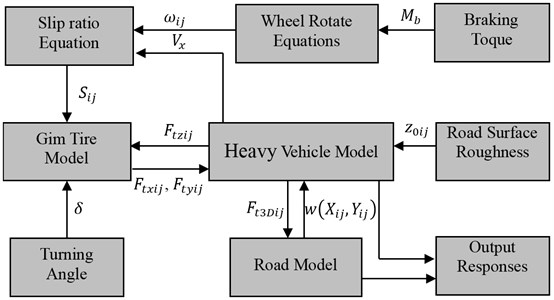As a comparison, the vertical coupled and uncoupled vehicle-road model are also built. For the vertical coupled model, only the vertical tire forces act on the road and the right hand of Eq. (34) is expressed by:

35
${R}_{ij}={\int }_{0}^{L}{\int }_{0}^{B}{\sum }_{i=1}^{3}{\sum }_{j=1}^{2}{F}_{t3Dij}\mathrm{s}\mathrm{i}\mathrm{n}\left(\frac{i\pi X}{L}\right)\mathrm{s}\mathrm{i}\mathrm{n}\left(\frac{j\pi Y}{B}\right)dXdY$
$\mathrm{}\mathrm{}\mathrm{}\mathrm{}\mathrm{}\mathrm{}={\sum }_{i=1}^{3}{\sum }_{j=1}^{2}{F}_{tZij}\mathrm{s}\mathrm{i}\mathrm{n}\left(\frac{i\pi {X}_{tij}}{L}\right)\mathrm{s}\mathrm{i}\mathrm{n}\left(\frac{j\pi {Y}_{tij}}{B}\right).$

For the uncoupled model, the vertical tire force doesn’t consider the effect of road vibration and is given by:

36
${F}_{tzij}={K}_{tij}\left({z}_{0ij}-{z}_{tij}\right)+{C}_{tij}\left({\stackrel{˙}{z}}_{0ij}-{\stackrel{˙}{z}}_{tij}\right)+\epsilon {K}_{tij}\left({z}_{0ij}-{z}_{tij}{\right)}^{2}.$

## 3. Comparison of responses of 3D coupled, vertical coupled and uncoupled vehicle-road models

In order to analyze the vehicle-road interaction using this proposed 3D coupled vehicle-road model, the computation method and the vehicle-pavement coupled model should be verified. However, it is very difficult to measure the vehicle and road dynamic responses simultaneously when a vehicle running on a road, especially when the vehicle turning, making a lane-change or braking. Due to lack of the test data of vehicle-road dynamics on every driving situation same to the simulation, only some simulation results were validated by experiments. Limited by the technical and economic conditions, we only obtained the test data of vehicle responses when the vehicle running at a constant speed along a straight line. By comparing with this test data, the validation of the 3D-coupled vehicle model has been given in the author’s another work . In order to add confidence to the validity the road model, the road responses simulated by the vehicle-pavement coupled model was compared with the simulation results of Wu , using the same parameters and boundaries conditions to Wu’s model. The comparison results were given in the author’s previous work .

As many scholars think, simulation is also a useful and economic method to research vehicle and road dynamics when the driving situations are difficult or dangerous to achieve. Compared with the analytical method, the simulation method is also convenient and flexible to discuss the engineering problem modeled by high-dimensional equations. As follows, the property of 3D vehicle-road interaction will be simulated in different driving situations.

Parameters for the heavy vehicle system are chosen according to a heavy truck with full load which was used in the field test :

${M}_{c}=$1115 kg, ${M}_{b}=$15797 kg, $M=$ 20440 kg, ${I}_{z}=$136×103 kg m2, ${l}_{1}=$5.20 m, ${l}_{2}=$1.15 m, ${l}_{3}=$1.3 m, ${l}_{4}=$4.83 m, ${l}_{5}=$ 1.2 m, ${l}_{6}=$1.0 m, ${L}_{s}=$0.778 m, ${H}_{s}=$0.52 m, ${K}_{sij}=$997.5 kN/m, ${C}_{sij}=$4000 N∙s/m, ${K}_{tij}=$2200 kN/m, ${C}_{tij}=$6300 N∙s/m, ${K}_{xij}=$373.8 kN/m, ${K}_{\alpha ij}=$454.6 kN/m ($i=$2, 3, $j=$1, 2), $\epsilon =$0.1, ${d}_{t1}={d}_{t2}=$1.9 m, $R=$0.42 m, ${\mu }_{0}=$0.01, ${\mu }_{1}=$0.9, ${S}_{1}=$0.15, ${K}_{c1}={K}_{c2}=$74.9 kN/m, ${K}_{c3}={K}_{c4}=$44.6 kN/m, ${C}_{c1}={C}_{c2}=$1985 N∙s/m, ${C}_{c3}={C}_{c4}=$1185 N∙s/m, ${K}_{s11}={K}_{s12}=$251.38 kN/m, ${C}_{s11}={C}_{s12}=$40 kN∙s/m, ${K}_{t11}={K}_{t12}=$1100 kN/m, ${C}_{t11}={C}_{t12}=$3500 N∙s/m, ${K}_{x11}={K}_{x12}=$186.9 kN/m, ${K}_{\alpha 11}={K}_{\alpha 12}=$227.3 kN/m.

Parameters for the pavement and foundation are chosen as follows [30, 31]:

${h}_{1}=$0.1 m, ${\mu }_{1}=$0.25, ${E}_{1}=$1600 MPa, ${\rho }_{1}=$2.5×103 kg/m3, ${h}_{2}=$0.2 m, ${E}_{2}=$700 MPa, ${\mu }_{2}=$0.25, ${\rho }_{2}=$2.2×103 kg/m3, $K=$48×106 N/m3, $C=$0.3×106 N∙s/m2, ${V}_{0}=$15 m/s, $L=$95 m, $B=$15 m. The random road roughness is B-class according to GB/T 7031-2005/ISO 8608:1995  and is generated using the method of Liu .

Four driving maneuvers are simulated, including straight-line driving at a constant speed, straight-line braking, and curve driving at a constant speed and combined steering and braking. By comparing the responses of the road and vehicle obtained by 3D coupled, vertical coupled and uncoupled models, the effects of coupling action on responses are analyzed.

Fig. 4Pavement displacements when straight-line driving at Vx= 15 m/s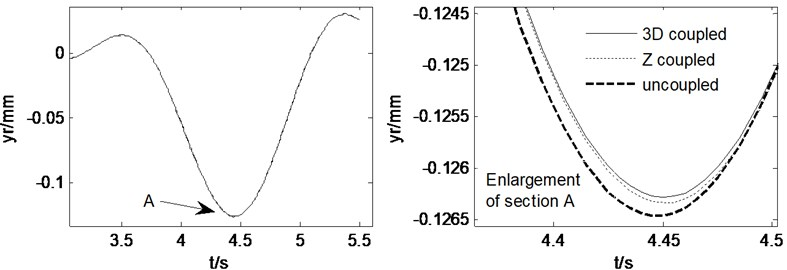Fig. 5Vehicle responses when straight-line driving at Vx= 15 m/s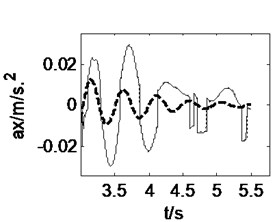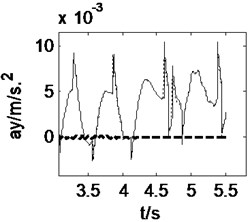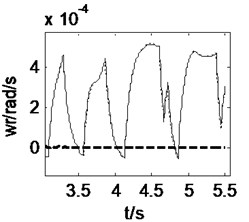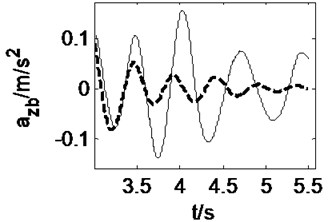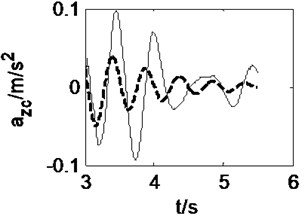### 3.1. Straight-line driving at a constant speed

Letting the heavy vehicle runs along a straight-line at a constant speed of 15 m/s, the pavement displacements and vehicle responses induced by tire forces can be obtained from three models, 3D coupled, vertical coupled ($Z$ coupled) and uncoupled vehicle-road models, as shown in Fig. 4 and Fig. 5. It may be noticed from Fig. 4 that the difference between 3D coupled model and $Z$ coupled model is slight and the pavement displacement decreases 0.2 % after considering the vehicle-road coupling action. In these three models, the 3D coupled model leads to the smallest pavement displacement. Fig. 5 shows that the effects of vehicle-road coupling on vehicle responses are great, but the difference between 3D coupled model and $Z$ coupled model is still slight. After considering the vehicle-road coupling action, the peak value of longitudinal acceleration of vehicle body, vertical accelerations of vehicle body and cab increases 275 %, 204 % and 145 % respectively. Both 3D and vertical vehicle-road coupling also lead to obvious increase of vehicle lateral acceleration and yaw rate. However, in the case of straight-line driving, the lateral acceleration and yaw rate of the vehicle are too small to be considered.

Fig. 6Pavement displacements when straight-line braking with Mz= –56500 N∙m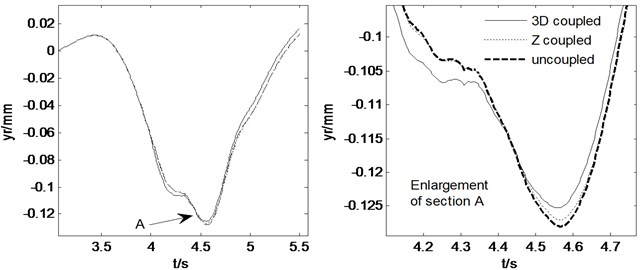Fig. 7Vehicle responses when straight-line braking with Mz= –56500 N∙m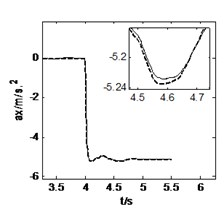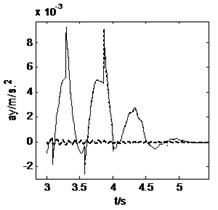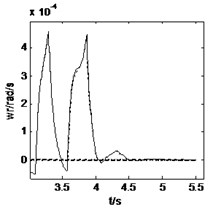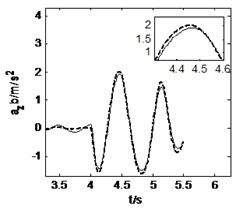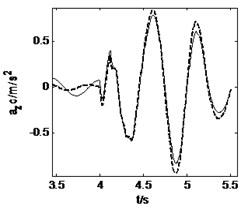### 3.2. Straight-line braking

The vehicle moves along a straight-line at an initial speed of 15 m/s and is braked with a braking torque ${M}_{z}=$56500 N∙m at the time of $t=$4 s. The road and vehicle responses are calculated from three models, as shown in Fig. 6 and Fig. 7. It can be obtained from these two figures that the 3D and vertical coupling action leads to 2.1 % and 0.8 % decrease of the pavement displacement respectively. Hence, when the vehicle is braking, the influence of 3D vehicle-road coupling action on pavement displacement is enlarged. In addition, the vehicle response difference between 3D coupled model and $Z$ coupled model is also very little. Compared with the uncoupled model, the two coupled vehicle-road models result in the peak value of longitudinal acceleration of vehicle body, and vertical accelerations of vehicle body and cab decreases 0.2 %, 5.0 % and 7.2 % respectively. It should be noted that the vehicle-road coupling causes a contrary impact on longitudinal vertical vehicle responses when vehicle is braking. However, the effects of vehicle-road coupling on the lateral acceleration and yaw rate are similar so long as the vehicle runs along a straight-line.

Fig. 8Pavement displacements when curving driving with δ= 0.15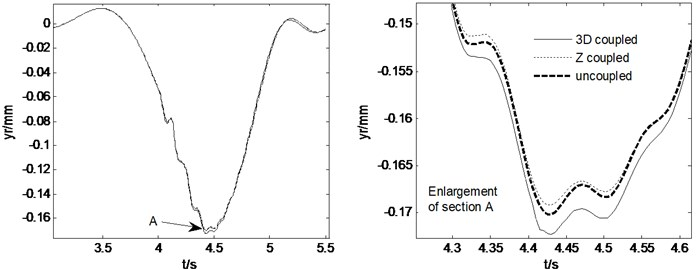Fig. 9Vehicle responses when curve driving with δ= 0.15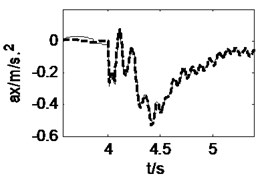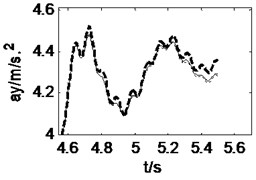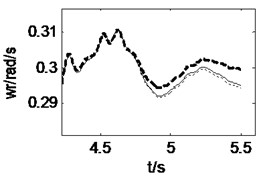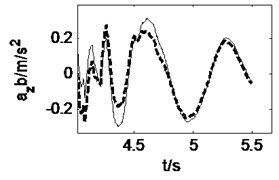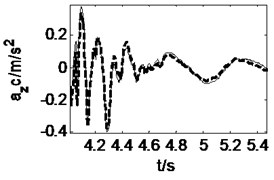### 3.3. Curve driving at a constant speed

When the vehicle is running along a straight-line at a constant speed of 15 m/s, a front wheel steering angle of 0.15 rad is input at $t=$4 s, and then the curve driving begins. The pavement displacements and vehicle responses are calculated using 3D coupled, vertical coupled and uncoupled vehicle-road models respectively, as shown in Fig. 8 and Fig. 9. It can be seen from Fig. 8 that the 3D and vertical coupling action result in 1.2 % and –0.7 % variation of the pavement displacement. It is interesting that the effect of vertical vehicle-road coupling action on pavement displacement is the same as the case of straight-line driving, but the effect of 3D vehicle-road coupling action is contrary. It may be implied that the influence of lateral tire forces on pavement displacement is contrary to that of vertical and longitudinal tire forces. Fig. 9 shows that the vehicle response difference between 3D coupled model and $Z$ coupled model is also tiny. With the coupling action considered, the peak value of longitudinal and vertical accelerations of vehicle body, the vertical accelerations, the lateral acceleration and yaw rate of cab changes –3.8 %, +31.2 %, +15.1 %, –0.9 %, +0.03 % respectively. Compared with the condition of straight-line driving at a constant speed, it is found that curve driving will enhance the influence of vehicle-road coupling on lateral vehicle responses.

Fig. 10Pavement displacements when combined steering and braking with δ= 0.15, Mz= –56500 N∙m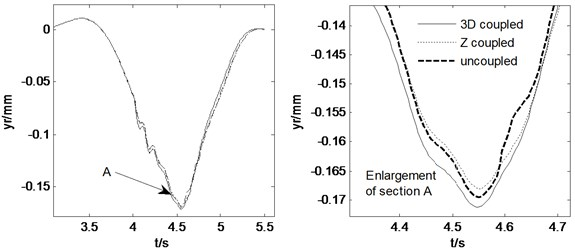Fig. 11Vehicle responses when combined steering and braking with δ= 0.15, Mz= –56500 N∙m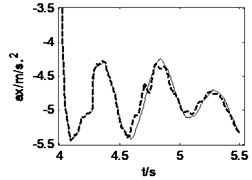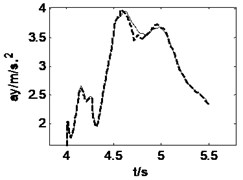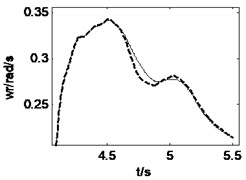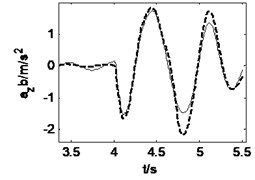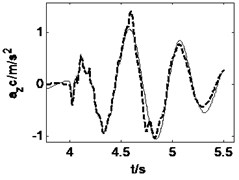### 3.4. Combined steering and braking

During the combined steering and braking driving maneuver, the heavy vehicle is input a front wheel steering angle $\delta =$0.15 and a braking torque ${M}_{z}=$–56500 N∙m simultaneously when $t=$4 s. The road roughness is B-class and the initial vehicle speed is 15 m/s. Fig. 10 and Fig. 11 show the road and vehicle responses obtained by the three vehicle-road models. It can be found from Fig. 10 that the 3D and vertical coupling action cause 1.1 % and –0.9 % change of the pavement displacement, and the pavement displacement obtained by 3D coupled model is the greatest. It should be noted again that the braking causes the decrease of the variation ratio. In addition, the vehicle response difference between 3D coupled model and $Z$ coupled model is also tiny. With the coupling action considered, the peak value of longitudinal acceleration, vertical accelerations of vehicle body and cab, lateral acceleration and yaw rate increases 0.2 %, –20.6 %, –24.3 %, –0.6 %, –0.3 % respectively. Therefore, in the combined steering and braking driving maneuver, the effect of vehicle-road coupling arrives at obvious impacts on three-directional vehicle responses and can’t be neglected.

By comparing the results of above four driving conditions, it may be summarized that:

1) In different driving conditions, the vehicle response difference between 3D coupled model and $Z$ coupled model is always tiny, but the difference between coupled and uncoupled model is too big to be neglected. Therefore, both 3D coupled model and vertical coupled model are good enough to predict vehicle responses accurately.

2) In straight-line driving condition, the pavement displacement obtained from 3D coupled model is smaller than that from vertical coupled and uncoupled model. But in curve driving condition, the pavement displacement predicted by 3D coupled model is bigger than those by the other two models. Whether in straight-line or curve driving condition, the vertical coupled model always obtains a smaller pavement displacement than the uncoupled model. Hence, the 3D coupled model is the most suitable for calculating road responses accurately.

## 4. Effects of system parameters on 3D vehicle-road interaction

When a vehicle runs on a road, it may meet a lot of vehicle driving conditions and road type. Thus it is necessary to research the effects of road and vehicle parameters on vehicle-road interaction. Since the effects of vehicle and road parameters on vertical vehicle-road interaction have been given in detail by the authors’ previous works [30-32], this work only puts emphasis on the effects of parameters on 3D vehicle-road interaction. During simulation, six parameters are selected, including the front wheel steering angle, the braking torque, the nonlinear coefficient of vertical tire stiffness, the vehicle running speed, the road surface roughness and the road type. The pavement displacement peak value and the difference between the results of 3D coupled model and vertical coupled model are calculated with different values of parameters, as shown in Figs. 12-15.

Fig. 12 shows that the rise of steering angle will increase amplitude of the pavement displacement, but a big braking torque will reduce the amplitude of pavement displacement. With the increase of front wheel steering angle or braking torque, the result difference between 3D coupled model and vertical coupled model also increases. Thus, during the maneuver of sharp steering or emergency braking, the role of 3D vehicle-road interaction is enhanced.

Fig. 12Effects of front tire turning angle and braking torque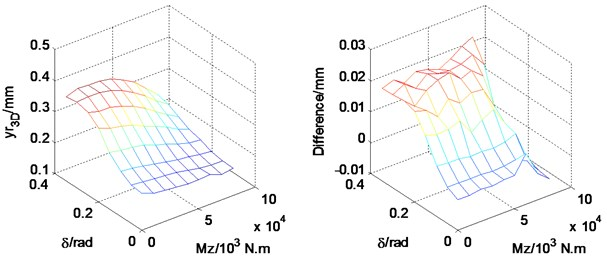From Fig. 13, it can be seen that a high vehicle speed increases the amplitude of pavement displacement. At the vehicle speeds higher than 60 km/h, the effect of the nonlinear tire coefficient increases substantially. In addition, with the increase of the vehicle speed or the nonlinear tire coefficient, the difference between 3D coupled model and vertical coupled model increases but is accompanied by occasional fluctuation. At the vehicle speeds higher than 60 km/h, the effect of the nonlinear tire coefficient increases substantially. Thus the nonlinear tire stiffness should not be neglected at higher vehicle speeds.

Fig. 13Effects of square nonlinear coefficient of tire stiffness and vehicle running speed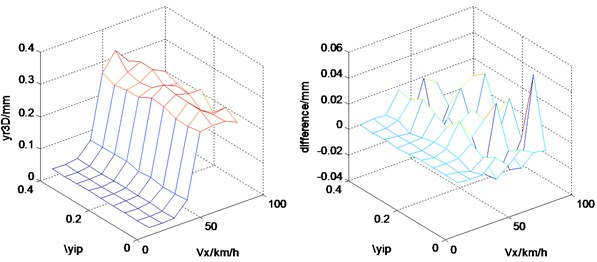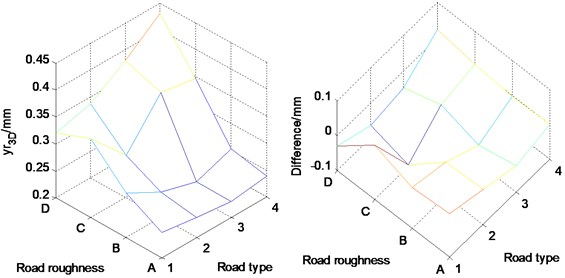## 5. Conclusions

An innovative three-directional coupled vehicle-road model is built for studying the properties of three-directional (3D) interaction between vehicle and road. It has been found that:

1) Both the 3D coupled model and the vertical coupled model are good enough to predict vehicle responses accurately, but the 3D coupled model is the most suitable for calculating road responses accurately.

2) During the maneuver of sharp steering or emergency braking, the road response difference between 3D coupled model and $Z$ coupled model is enhanced and the 3D vehicle-road interaction should be considered.

3) When a vehicle runs on a road with small surface roughness and big adhesion coefficient, the difference between results of 3D coupled model and vertical coupled model is obvious and the 3D vehicle-road interaction should be considered.

4) With the increase of the vehicle speed or the nonlinear tire coefficient, the difference between 3D coupled model and vertical coupled model increases but is accompanied by occasional fluctuation. The nonlinear tire stiffness should not be neglected at higher vehicle speeds.

In this work, the value of tire stiffness is selected as to the normal air pressure in the tire and the square nonlinear tire model is applied. In fact, a finite element tire model can consider the effect of air pressure in the tire and the tire structure better. However, how to connect a finite element tire model with a vehicle model and a road model is still a challenging problem, which is also our future research direction. In addition, the vehicle-road tests on complicated driving situations including lane change, turning and braking need to be fulfilled so as to validate the simulation results.

23 July 2015
Accepted
06 October 2015
Published
15 November 2015
SUBJECTS
Vibration in transportation engineering
Keywords
heavy vehicle